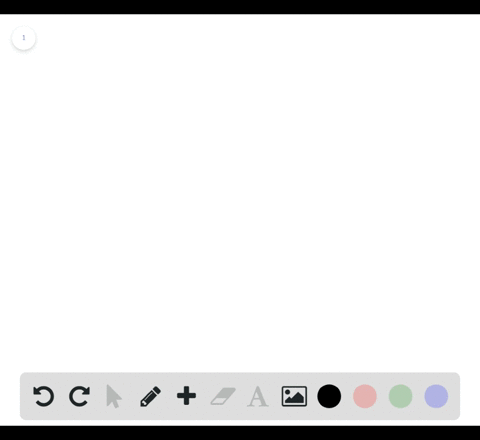Enroll in one of our FREE online STEM bootcamps. Join today and start acing your classes!View Bootcamps02:03
Problem 50

# Using the $\varepsilon$ -\delta Definition of Limit In Exercises $45-56$ , find the limit $L$ . Then use the $\varepsilon-\delta$ definition to prove that the limit is $L$ .$$\lim _{x \rightarrow 2}(-1)$$

## Discussion

You must be signed in to discuss.

## Video Transcript

and this problem we want to find the limit is ex Purchase two off. The constant function f of X equals negative one. So it can't have that re written right here. So our post answer is that the limits equal to make one. So if you kind of graph that function, you'll see that you have a horizontal line located at negative one. So actually the limit is X approaches. Any real number for this function will be negative one. So that's kind of thie intuition behind thiss proposed answer here. We want to show this using an absolute delta proof that is, we want to show that for every Absalon greater than zero, there is a delta greater than zero such that negative one minus negative one is less than epsilon. Whenever zero is less than the absolute value of X minus two is less than don't. So, first, we should note that if we have no the absolute value of negative one minus negative one, it's less than absolutely then if we simplify that we have that zero in absolute value is less than Epsilon. And we can write that because the up survive. Sarah was only Cero. So that leaves Ciro is less then. Absalon. So this may look a little strange, but we'll see that our problems became a lot more simple here. Because remember, we have this found up here, two on the absolute value of X minus two. We don't know that that's between zero and Delta. So that right here and say this definitely implies that Ciro is less than daughter if we kind of take out this middle part. So if we let Absalon Keyhole to doubt her Yeah, then we have thie. Absolute value of negative one, Linus. Negative one, which is equal too. So which is certainly us, then Delta, which is he called to Absalon. And we're done. Yeah.